# Numbers - math word problems

#### Number of problems found: 2341

• Age ratioJanko is 14 years old. The age ratio of Janka and Zuzka is 2: 3. What was the ratio seven years ago?
• Negative difference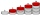Find four arithmetic progression members between 7 and -6.
• AP membersWhat is the value of x2, x3, x4, x5…of the terms (of arithmetic progression) when x1 = 8 and x6 = 20?
• Group of childrenThere is a group of children. There is a boy named Adam in each of the three children subgroup and a girl named Beata in each quartet (four-member subgroup). How many children can be in such a group and what are their names in that case?
• On the number lineWhat number is exactly in the middle between 9.8 and -2.4 on the number line?
• Prospering studentsThere are girls and 30 boys in the class. Boys prospering 28, girls all. How many girls are in the class and how many boys are there, if all the prospering students are 95%?
• Solve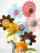Solve this direct relation by the triplet: 0.25h. .. . . .. .. . . .. .. .. 3 pcs 6.5h. .. . . .. .. . . .. .. . . .. .. x pcs
• Row for lunchThere are less than 66 people in a row for lunch. In front of Eva, there are 28 students. Behind Martin, there are 30 people. Between Eva and Martin, there are 17 people. How many students stand behind Eva if Martin stands in front of Eva?
• Basements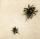In the first basement is more flies than the spiders, the second vice versa. Each basement had spiders and flies together 100 feet. Determine how many could be flies and spiders in the first and second basement. PS. We only need, when you write how many s
• MisterMister ignacio paid Php.13693.75 for a television set that originally cost Php.15,650 . Find the rate of discount. Please show your solution please please please.
• Wire D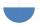Wire length 1 m is bent so that it forms a semicircle circuit (including the diameter). Determine the radius of the semicircle.
• StudentsIf the fifth-grade class left 20% of students and in the seventh grade were added two pupils, in the eighth one pupil, the number has not changed in the ninth grade, but it is now tenth students less than it was in the fifth grade. How many pupils are in
• The dough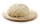The dough contains water, flour, and sugar. Water and flour in a ratio of 2: 3, flour and sugar in a 2: 1. Find the ratio of all three components of the dough.
• Profitable companyThree businessman decide to open up their own company. They agree to distribute the yearly profits made in the same ratio as their initial investments. They invest R 50 000, R 75 000 and R25 000, respectively. The profit made by the company in the first y
• Right key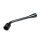The hostel has 4 rooms. The keys to each room are not numbered. Each of the four guests took one key. What is the probability that everyone took the right key?
• Balls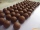From the bag with numbered balls (numbers 1,2,3,. ..20) we pick one ball. What is the probability of choosing a number containing 1?
• PagansElena cut out same circle-shaped pagans and put them on a rectangular sheet so that the neighboring pagans were touching each other and the pagans were touching the walls of the sheet on the edges. Each pagans occupied 28.26 cm2 of the bottom of the sheet
• Exponential equationSolve exponential equation (in real numbers): 98x-2=9
• The number 72The number 72 increase by 25%. By how much % will you have to reduce the number you created to get the number 72 again?
• Division of moneyCalculate how many euros have Matthew, Miriam, Lucy, Michael, Janka when together have 2,700 euros and the amounts are at a ratio of 1:5:6:7:8.

Do you have an exciting math question or word problem that you can't solve? Ask a question or post a math problem, and we can try to solve it.

We will send a solution to your e-mail address. Solved examples are also published here. Please enter the e-mail correctly and check whether you don't have a full mailbox.